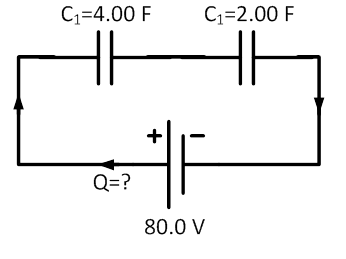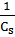### Sample Problem

In the following circuit, what is the charge of the 2.00 F capacitor?#### Solution

Use the equation= 1/2 + 1/4 = 3/4

Cs = 4/3 = 1.33 F

C = Q/V

Qtotal = CV = 1.33 F × 80.0 V = 106 C

Since Qtotal = Q1 = Q2… total charge is the same for each capacitor, the charge for the 2.00 F capacitor is 106 C